Why monopolies are bad for consumers: a math example using marginal revenue, part 1 - FreeEconHelp.com, Learning Economics... Solved!

## 6/27/11

In today's post I will go over the basic economic interpretations for a monopoly and explain why a monopoly holds market power and what this means for the consumer.  First it is important to recognize that monopolies only have power if they are the only seller of a good or service, and there are no close substitutes, check out this most for more information on the definition of a monopoly.

Because there are no other options for consumers to choose from, if they want the good, they have to buy it from the monopoly.  Because they have little choice, monopolies
get to choose how much they sell, and based on the associated demand functions, they know how their choice of quantity will affect price.  An example of this demand equation is shown below: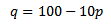This shows what the quantity demanded will be give a price.  However, for the monopolist, we want to know what price will be given a choice of quantity.  So by solving for p we get: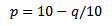So the monopoly faces a trade off here, if they decide to sell more of their good, then they have to do so at a lower price.  If they decide to raise the price, then they have to sell less goods.  So the monopoly wants to find out at what point they can maximize their own profit, by manipulating prices and quantities.  The easiest way to look at this is through the introduction of marginal revenue (MR) for monopolies.

To calculate the marginal revenue for the monopoly, we first have to calculate what the total revenue is. This is done for us in the table below by multiplying the quantity value times the price.  We know the relationship between these two variables because they are based on the functions described before.  To get the marginal revenue, we simply subtract the previous total revenue from the current total revenue.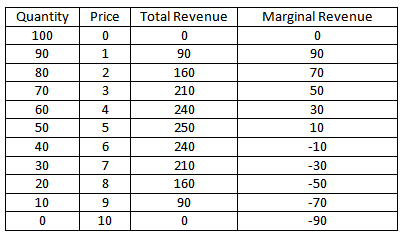This table shows us two relationships that we should remember when analyzing monopolies.  The first is that the slope of the marginal revenue curve is always twice as steep as the demand curve.  We can see this easily in the table above because the marginal revenue is decreasing by 20 for each price increase, while the quantity demanded is only decreasing by 10.  The second is that the marginal revenue curve always intersects the origin halfway between the max and min quantity demanded (which is related to the twice slope idea mentioned above).  Here it occurs at 50, as we would expect.  A graphical representation of a monopoly and its marginal revenue curve is shown below: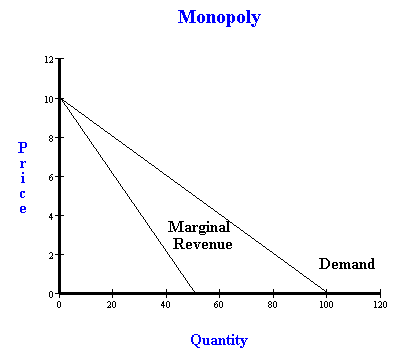But why is this the case?  It has to deal with elasticities, and where consumers have elastic price elasticities of demand or not.  When the monopoly can increase its revenue by lowering price, than consumers have an elastic price elasticity of demand, however when less revenue is made by lowering the price, then they are operating in the inelastic price elasticity of demand region.  This relationship is shown below: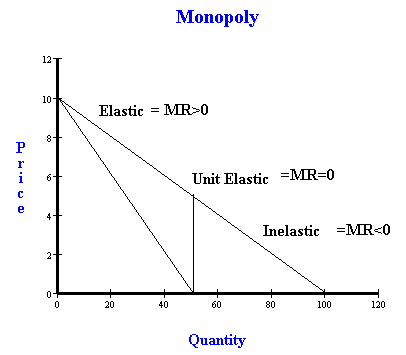Remember:  The slope of the marginal revenue line is always twice that of the demand curve.  And that the marginal revenue line always intersects the X axis at the halfway point where the demand curve does, and this will be at the point of unit price elasticity.